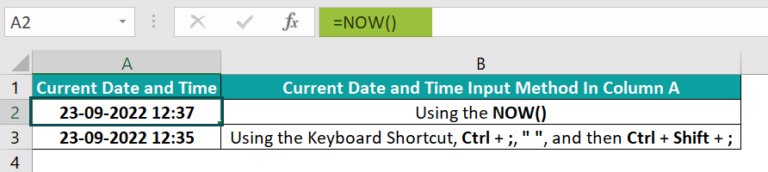# NOW Function In ExcelArticle byExcelMojo Team## What Is NOW Function In Excel?

The NOW function in Excel is an inbuilt Date & Time function that displays the current date and time. And the output gets updated every time we open or refresh the workbook. Users can use the NOW() when determining values based on the current date and time. The function is useful while preparing dynamic KPI reports for financial analysis.

For example, the below table shows the requirements in column A. Now, we need to display the required date and time in column B.

Using NOW function in Excel, we can update column B with the required data.

The above example shows the specific duration getting deducted from or added to the NOW() output. It depends on whether the required current date and time is a past or future value.

Likewise, we can use the NOW function in Excel with other Excel functions, such as IF, DAYS, TIME, and INT, to make the most of it.

###### Key Takeaways
• The NOW function in Excel returns the current system date and time as a dynamic value, which is useful while preparing KPI reports for financial analysis.
• The NOW() does not take any arguments as input.
• We can enter the NOW() by:
• Using the option Formulas 🡪 Date & Time 🡪 NOW.
• Manually entering the NOW() in the target cell.
• Using the keyboard shortcut, Ctrl + ;, ” “, and then Ctrl + Shift + ;.
• We can achieve impressive results using the NOW() with other Excel functions, such as IF, DAYS, and TIME.

### NOW() Excel Formula

The syntax for the NOW formula in Excel is:

Please Note: The NOW() does not take any arguments as input.

Below are a few critical aspects we should know while using NOW function in Excel.

• The NOW() returns the current date and time, as a dynamic value, based on the clock on our computer.
• If the target cell Number Format is General, the NOW() output will be the serial number representing the current date and time. On the other hand, if the Number Format is Date, the NOW() output will only show the current date. And with the Number Format custom set as date and time, the NOW() will show the current date and time.
• The NOW function is volatile. So, when we edit the worksheet or refresh or re-open the workbook, the function automatically recalculates and displays the refreshed NOW() output.
• We can also click anywhere in the active worksheet and press Shift + F9 to update the NOW function in Excel return value in the cell containing the NOW(). On the other hand, we will have to press F9 to update all our open workbooks.
• The time values get represented using decimal numbers, with the numbers denoting the days and the fractions denoting the hours.

### How To Use NOW Excel Function?

The steps to use the NOW function in Excel are:

1. Select the target cell where we want to display the current date and time.
2. Enter the NOW() and press Enter.

Let us see the steps with an example. Consider the table below and assume we need to update column A with the current date and time.

We can execute the NOW function in Excel using three ways to get the required data.

1. Select the target cell A2 and click Formulas 🡪 Date & Time 🡪 NOW to open the Function Arguments window.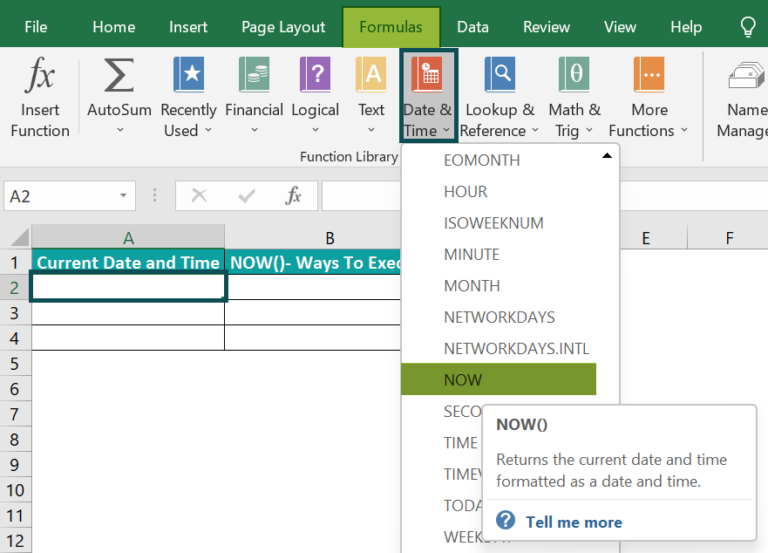Once we click on the NOW(), the below Function Arguments window will open, where we need to click OK.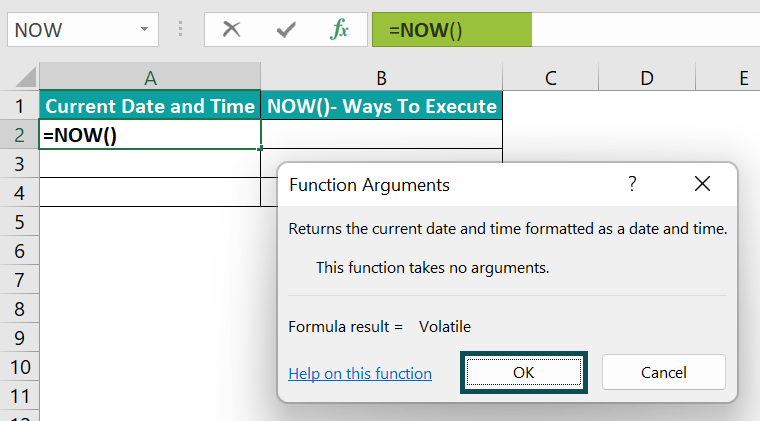The NOW function in excel return value in the target cell A2 will be: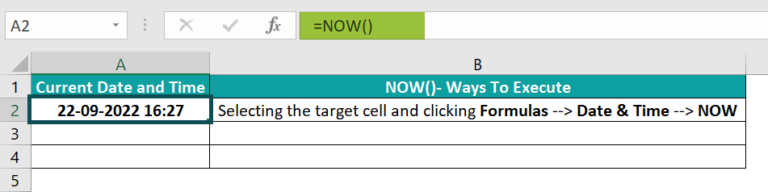2. Select the target cell A3, manually enter the NOW(), and press Enter.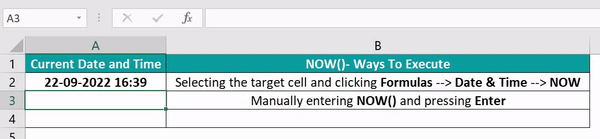3. Select the target cell A4 and first press Ctrl + ; to enter the current date. Then press the space bar to enter a space character. Finally, press Ctrl + Shift + ; to get the current time.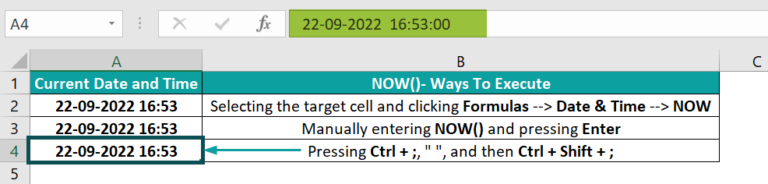The third technique uses the keyboard shortcut to enter the current date and time in the target cell. However, if we use it, the output does not update automatically every time we re-open or refresh the workbook.

### Examples

Here are some scenarios where we can apply the NOW function in Excel.

#### Example #1

The example below shows how we can use TODAY and NOW function in Excel to enter the current time without displaying the date. Also, the time will dynamically get updated each time we make changes to the spreadsheet or re-open it.

Suppose below is a table where we need to update the current time in cell B3, which will update automatically, the same way as when using the NOW function.

We can achieve the required result using the NOW and TODAY function in Excel, and the steps are:

Step 1: To begin with, select cell B1, enter the NOW(), and press Enter.

Step 2: Next, select cell B2, enter the TODAY(), and press Enter.

Step 3: Select the target cell B3, enter one of the below formulas, and press Enter.

=B1-B2 or =NOW() – TODAY()

Cell B3 contains the current time, but the Number Format is set as General, as highlighted in the above image.

We will have to change the Number Format to get the time in the proper format.

Step 4: Select cell B3 and set the Number Format in the Home tab as Time.

Alternatively, we can select cell B3 and click on the More Number Formats option in the Number Format drop-down list to open the Format Cells window.

In the Format Cells window, select the Custom option in the Number tab and choose the required time format, as depicted in the below image.

And once we click OK, the output in cell B3 will be:

So, when we set the proper time format, we can confirm the current time obtained in cell B3, using the functions TODAY and NOW, is the same as what the NOW() returned in cell B1.

#### Example #2

This example shows us how to use the NOW with the IF function in Excel.

Consider the below table. It shows project submission deadlines in column A.

Suppose we need to update the table with the current date, time, and project status. Then we can use the NOW function and the IF() to get the required data.

Step 1: To begin with, select cell B2, enter the NOW(), and press Enter.

Step 2: Drag the fill handle downwards to copy the formula in the cell range B3:B6.

Step 3: Select cell C2, enter the formula containing the NOW() and IF(), and press Enter.

Step 4: Drag the fill handle downwards to copy the formula in the range C3:C6.

The IF() checks whether the difference between the submission deadline and the current date and time is greater than 0. If the condition holds, the project is due for submission. Otherwise, the project missed the submission deadline.

#### Example #3

This example shows how to use the NOW function in Excel with other built-in functions such as DAYS().

Suppose the current date is 23-09-2022, and we have the leadership meeting schedules for the next three months.

And we require to check the number of days left for each meeting from the current date. Though the current time is not a criterion, we can apply the DAYS() and the NOW function in Excel to get the output.

The steps are:

Step 1: First, select the target cell C2, enter the below formula, and press Enter.

=DAYS(A2,NOW())

Step 2: Drag the fill handle downwards to copy the formula in the cell range C3:C7.

In the above formula, the NOW() returns the current date and time. And the DAYS() require two arguments as input, end_date and start_date.

So the DAYS() takes the dates in columns A and B as the end_date and start_date. It then computes the total number of days between the two specified dates to display as the output in each cell from the range C2:C7.

### Important Things To Note

• The NOW function in Excel, by default, displays the current date and time in the custom format, m/d/yyyy h:mm.
• Choose the proper data format from the Number Format options in the Home tab to display the NOW() return value in the required format.
• The NOW() is volatile. Every time we edit or open the worksheet, the function recalculates and returns the updated current date and time.
• When we apply the Excel keyboard shortcut, Ctrl + ;, a space character, Ctrl + Shift + ;, to enter the current date and time, the output will be static.

1. Where is the NOW function in Excel?

The NOW function in Excel is in the Formulas tab. We will have to click Formulas 🡪 Date & Time 🡪 NOW.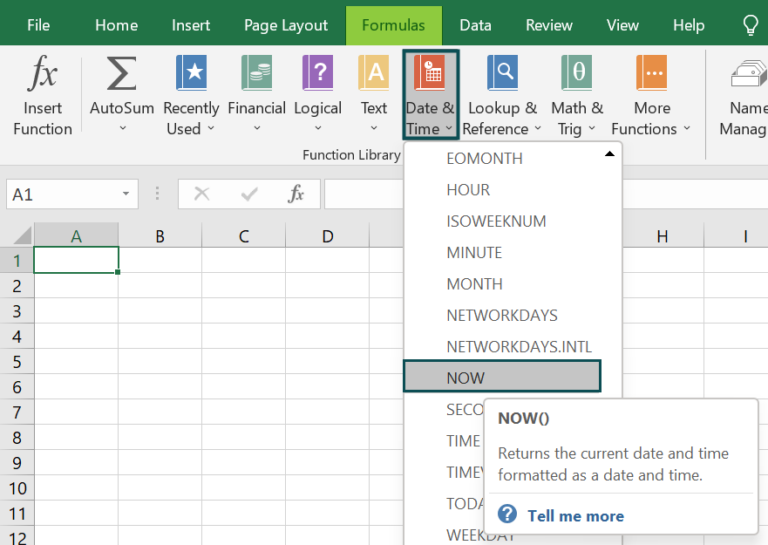2. What does the NOW() return in Excel?

The NOW() returns in Excel the current date and time. And the return value gets updated each time we make changes in our spreadsheet or open our workbook.

We can change the NOW() output data format to display only the date or time according to our requirements.

3. How do I make a static function NOW?

We can make a static function NOW using the below methods:

● Once we enter the NOW() in the target cell, press Ctrl + C to copy the function. And then press Alt + E + S + V one after the other to paste the value into the target cell without the formula. Thus, this method will display the cell content as a static value.
● Apply the keyboard shortcut keys to enter the current date and time in the target cell. First press Ctrl + ;, then a space character, and finally press Ctrl + Shift + ;. We will see the current date and time with a space in between.
Thus, using these techniques, we can use the NOW() to enter the current date and time. But these values will not get updated every time we make changes in the spreadsheet or open the workbook, thus making the NOW() behave like a static function.

4. Is NOW() volatile?

The NOW() is volatile. Every time we edit or re-open our spreadsheet, it recalculates and returns dynamic current date and time values.

For example, consider the below table.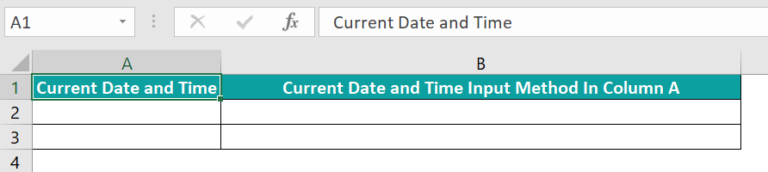Suppose we use the NOW() to enter the current date and time in cell A2.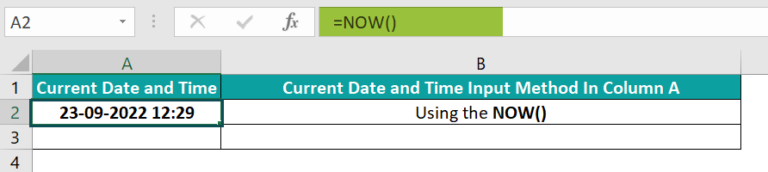And then, using the keyboard shortcut, Ctrl + ;, ” “, and then Ctrl + Shift + ;, we update the required value in cell A3.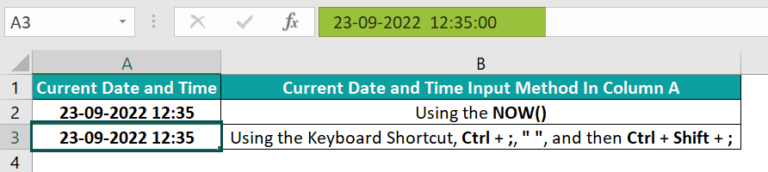Now, assume we save and close the worksheet and re-open it after a minute. Then, we will see the refreshed current date and time in cell A2, containing the NOW(), as depicted in the below image.